# Debt Schedule with PMT, IPMT and IF Formulas

Use Excel's PMT, IPMT, and IF formulas to create a debt schedule

## Debt Schedule with PMT, IPMT, and IF

We can use Excel’s PMT, IPMT, and IF formulas to create a debt schedule.  First, we need to set up the model by inputting some debt assumptions.  In this example, we assume the debt to be \$5,000,000, the payment term to be 5 years, and the interest rate to be 4.5%.

1. The opening balance in our debt schedule is equal to the loan amount of \$5 million, so in cell E29 we enter =B25 to link it to the assumption input.  Then, we can use the PMT formula to calculate the total payment for the first period =PMT(\$B\$27,\$B\$26,\$B\$25).  The formula calculates the payment amount using the loan amount, term, and interest rate stated in the assumption section.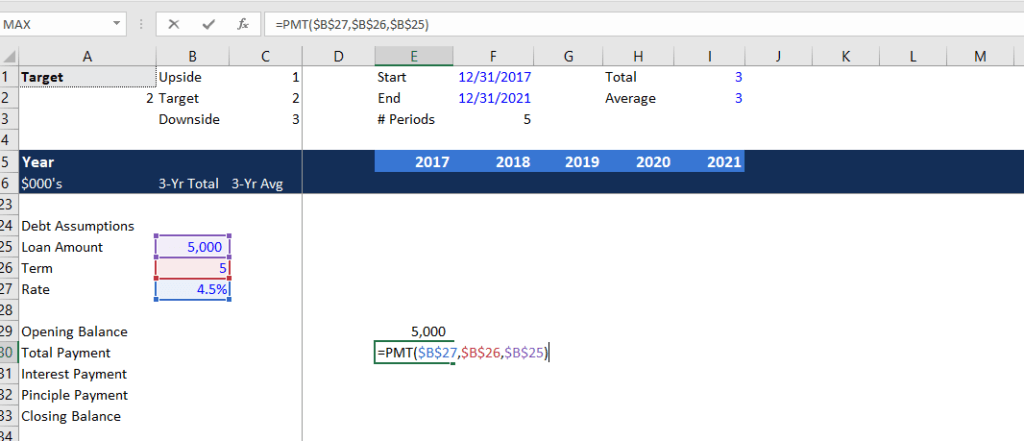2. In cell E28, input the period we are in, which is 1. In cell E29, enter =E28+1 and fill the formula to the right.  Next, use the IPMT formula to find out the interest payment for the first period =IPMT(\$B\$27,E28,\$B\$26,\$B\$25).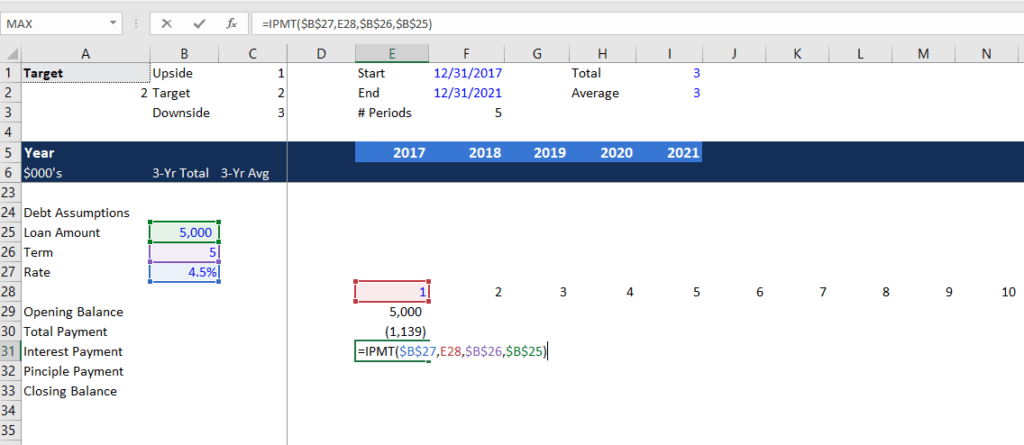3. The principal payment is the difference between total payment and interest payment, which is =E30-E31. The closing balance is the opening balance plus the principal payment being made, which is =E29+E32.  The opening balance for period 2 is the closing balance for period 1, which is =E33.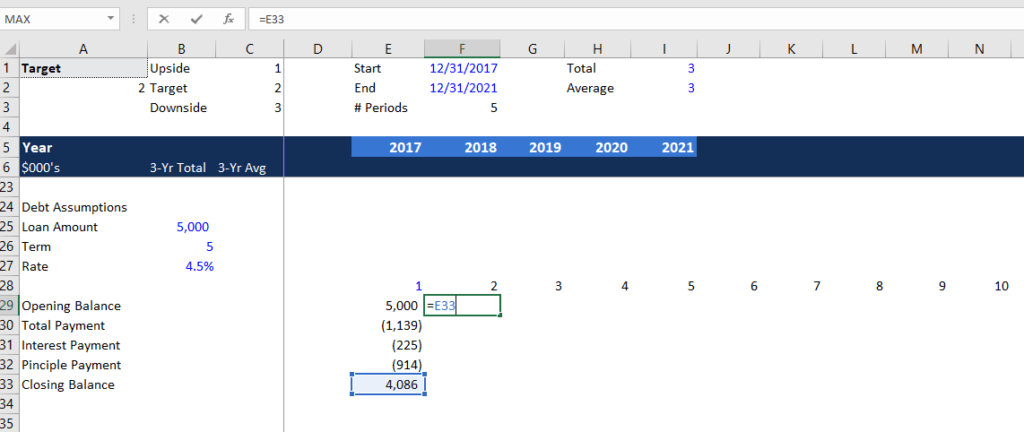4. Copy all formulas from cell E29 to E33 to the next column, then copy everything to the right. Check if the closing balance for period 5 = 0 to ensure correct formulas and numbers are being used.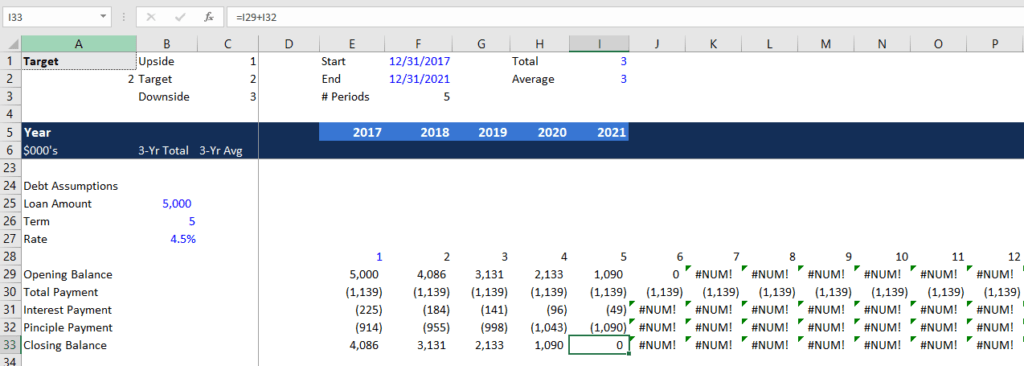5. Notice that there are some error messages starting from period 6 because the opening balance is 0. Here we can use the IF function to clean the errors.  In cell E30, type =IF(E29>0,PMT(\$B\$27,\$B\$26,\$B\$25),0).  The formula states that if the opening balance is less than 0, then the total payment value will be shown as 0.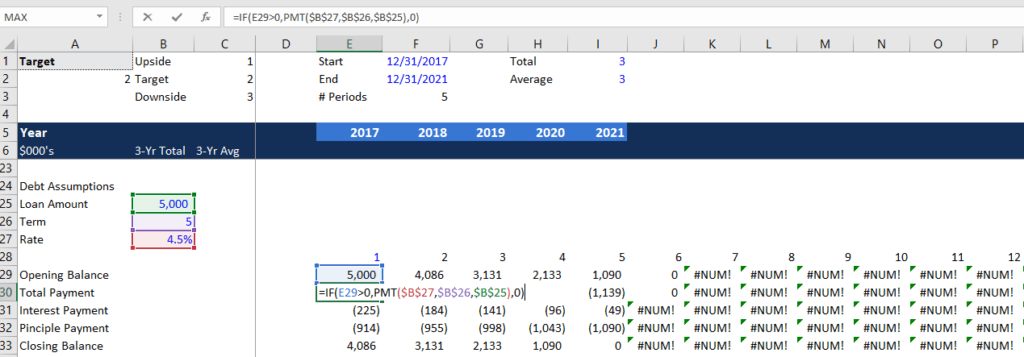6. In cell 31, type =IF(E29>0,IPMT(\$B\$27,E28,\$B\$26,\$B\$25),0). This formula is similar to the previous one, which states that if the opening balance is less than 0, then the interest payment will be shown as 0.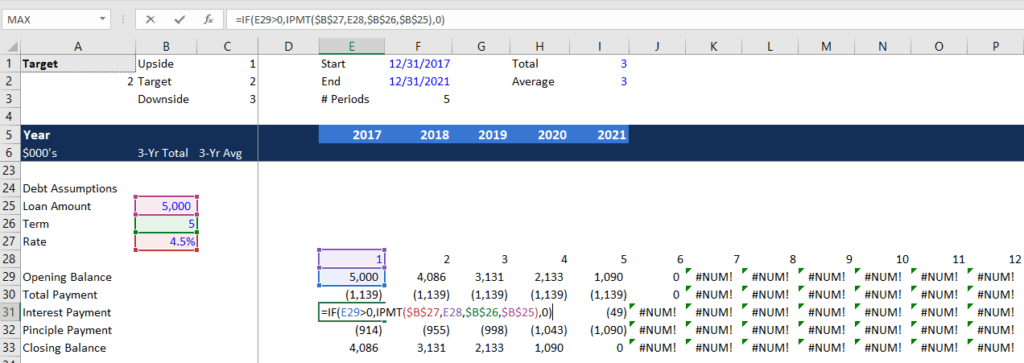7. Copy cell E30 and E31, press SHIFT + right arrow then CTRL + R to fill right. You should see that all the error messages are now shown as 0.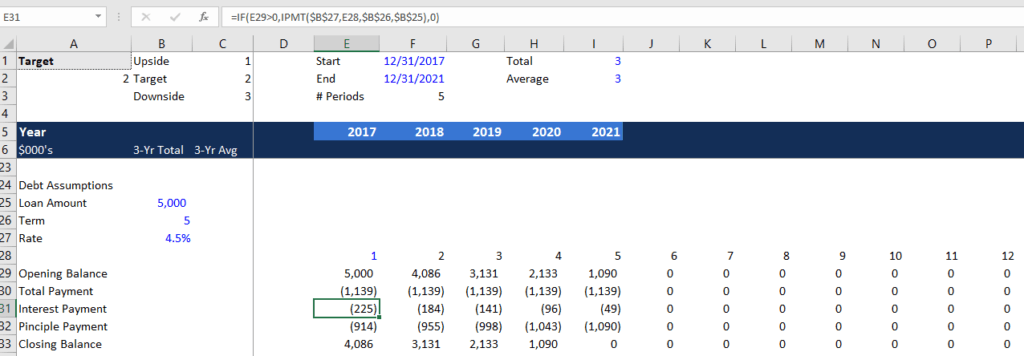### XNPV an XIRR with DATE and IF functions

We can calculate the NPV and IRR based on specific dates using the Excel functions XNPV and XIRR with the DATE and IF functions.

8. Go to cell E6 and enter =DATE(E5,12,31) to display the date. Copy to the right. You will see the #VALUE! message after 2021. We can fix this by using the IFERROR function =IFERROR(DATE(E5,12,31),””).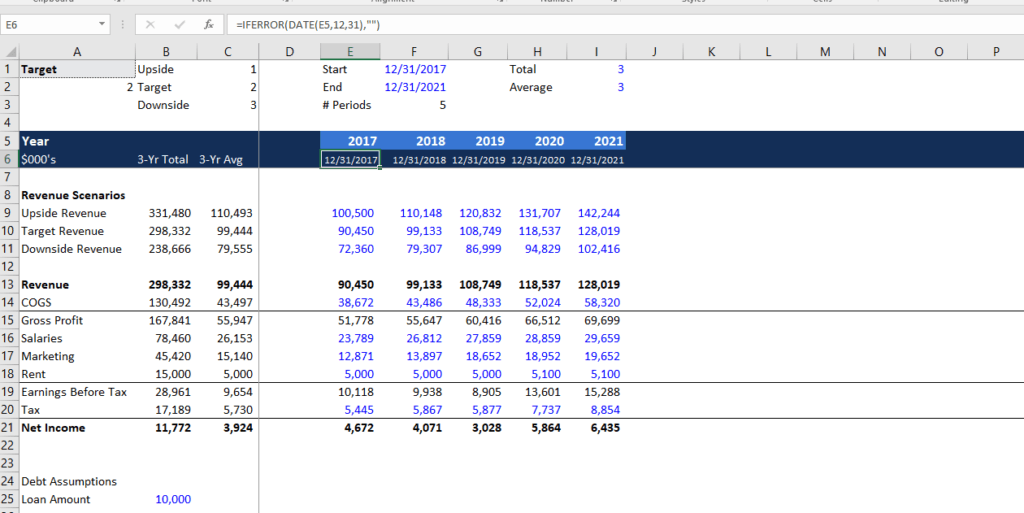9. Now we can begin to calculate the NPV and IRR. First, we need to input the free cash flow amounts. We assume that the FCF amounts from period 1 to 5 are -1,000, 500, 600, 700, 900.  In cell C37, we will enter a discount rate of 15%. In cell B37, calculate the NPV using the XNPV formula =XNPV(C37,E35:I35,E6:I6).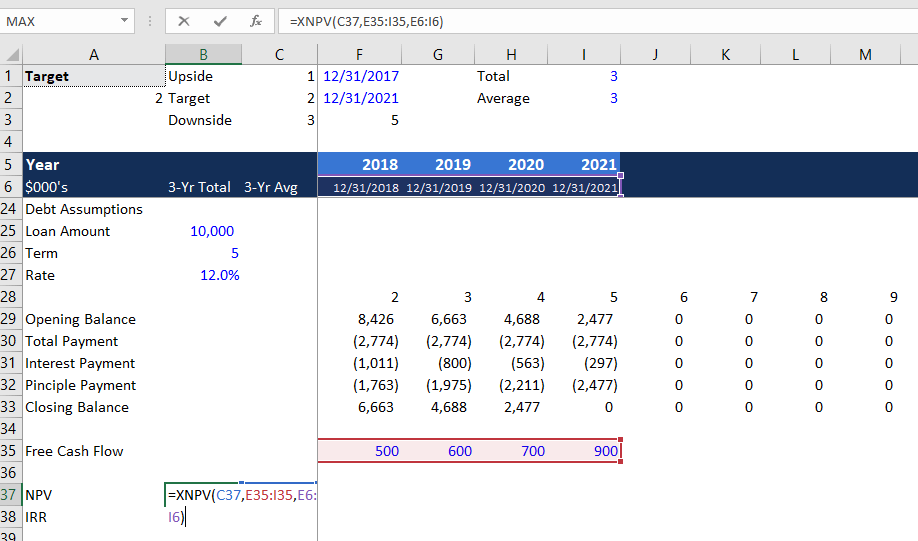10. In cell B38, calculate the IRR using XIRR formula =XIRR(E35:I35,E6:I6).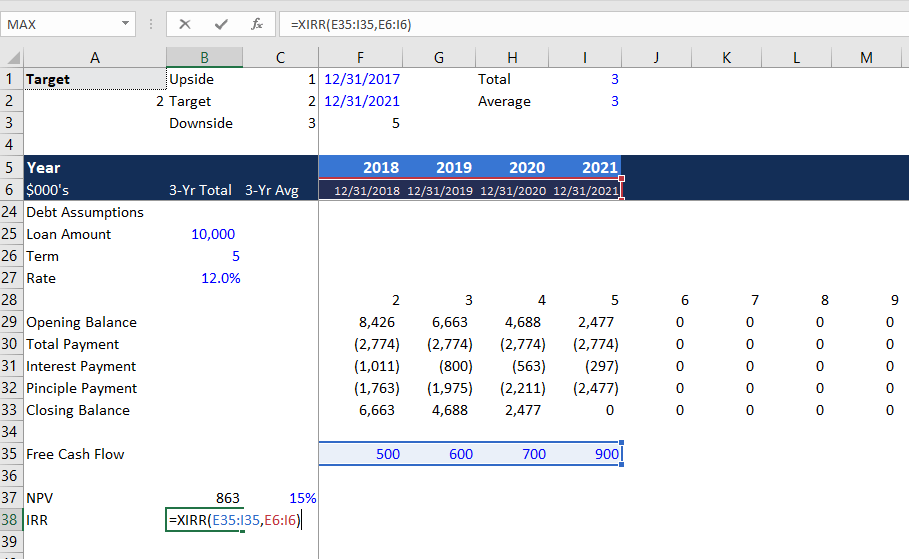### Adding OFFSET to XNPV and XIRR

We can change to the XNPV and XIRR formulas to make more dynamic formulas using the OFFSET function.

11. In cell B42, change the formula to =XNPV(C42,E40:OFFSET(E40,0,\$F\$3-1),E6:I6). The formula is more dynamic because if the number of periods increase, then the periods of free cash flow will also increase. We don’t need to change the NPV formula if the forecast period is longer. For the IRR function, change it to =XIRR(E40:OFFSET(E40,0,\$F\$3-1),E6:I6).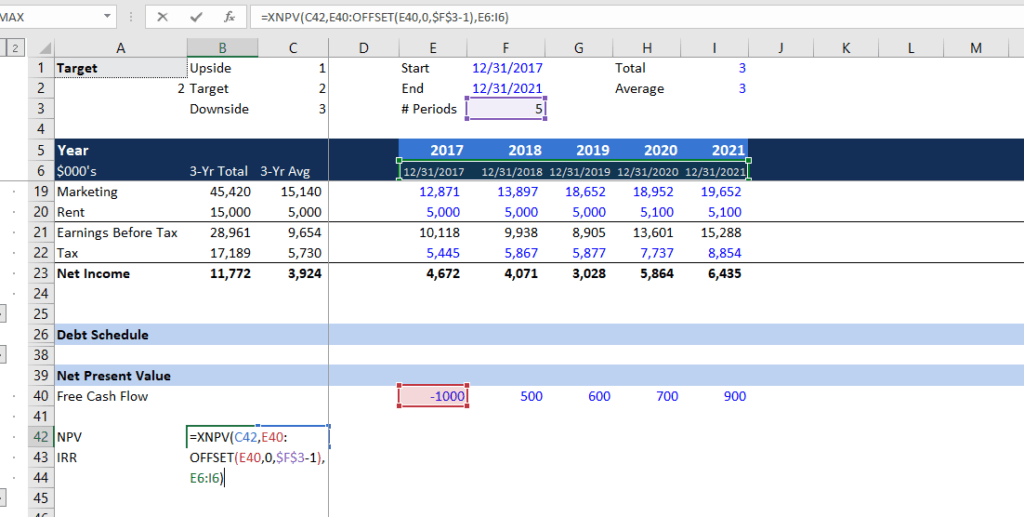12. After adjusting the formula for the number of periods, we should offset the dates. In cell B42, change the formula to =XNPV(C42,E40:OFFSET(E40,0,\$F\$3-1),E6:OFFSET(E6,0,\$F\$3-1)). This allows the NPV and IRR formulas to pick up the right number of free cash flow with the change in the number of periods.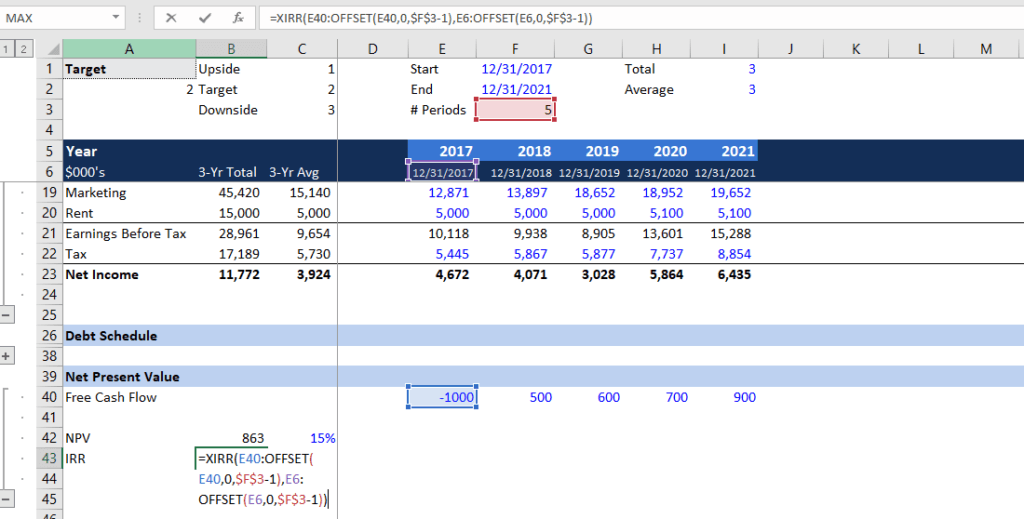### Summary of Key Debt Schedule Formulas

• PMT formula for calculating debt payment amount: =PMT(interest rate, number of terms, present value)
• IPMT formula for calculating interest payment: =IPMT(interest rate, period, number of terms, present value)
• XNPV formula for finding the net present value: =XNPV(discount rate, free cash flows, dates)
• XIRR formula for finding the internal rate of return: =XIRR(free cash flows, dates)
• OFFSET formula for calculating dynamic NPV: =XNPV(discount rate, 1st FCF : OFFSET(1st FCF, 0, # periods – 1), 1st date : OFFSET(1st date, 0, # periods – 1))
• OFFSET formula for calculating dynamic IRR: =XIRR(1st FCF : OFFSET(1st FCF, 0, # periods – 1), 1st date : OFFSET(1st date, 0, # periods – 1))

### Other Resources

Thank you for reading CFI’s guide on Debt Schedule with PMT, IPMT and IF formulas.  To keep learning and advancing your career, the following CFI resources will be helpful:

• Basic Excel Formulas
• Financial Modeling Best Practices
• List of Excel Functions
• Overview of Excel Shortcuts

### Free Excel Tutorial

To master the art of Excel, check out CFI’s FREE Excel Crash Course, which teaches you how to become an Excel power user.  Learn the most important formulas, functions, and shortcuts to become confident in your financial analysis.

Launch CFI’s Free Excel Course now to take your career to the next level and move up the ladder!